## Lal's Constant

Letdenote the number of Primes of the formfor, then(1)

where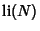is the Logarithmic Integral (Shanks 1960, pp. 321-332). Let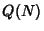denote the number of Primes of the form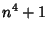for, then(2)

(Shanks 1961, 1962). Letdenote the number of pairs of Primes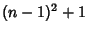andfor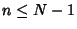, then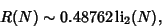(3)

where(4)

(Shanks 1960, pp. 201-203). Finally, letdenote the number of pairs of Primesandfor, then(5)

(Lal 1967), whereis called Lal's constant. Shanks (1967) showed that.

References

Lal, M. Primes of the Form.'' Math. Comput. 21, 245-247, 1967.

Shanks, D. On the Conjecture of Hardy and Littlewood Concerning the Number of Primes of the Form.'' Math. Comput. 14, 321-332, 1960.

Shanks, D. On Numbers of the Form.'' Math. Comput. 15, 186-189, 1961.

Shanks, D. Corrigendum to On the Conjecture of Hardy and Littlewood Concerning the Number of Primes of the Form.'' Math. Comput. 16, 513, 1962.

Shanks, D. Lal's Constant and Generalization.'' Math. Comput. 21, 705-707, 1967.